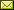## Deterministic Temporal Logics and Interval Constraints

 Kamal Lodaya (The Institute of Mathematical Sciences, Chennai) Paritosh K. Pandya (Tata Institute of Fundamental Research, Mumbai)

 In temporal logics, a central question is about the choice of modalities and their relative expressive power, in comparison to the complexity of decision problems such as satisfiability. In this tutorial, we will illustrate the study of such questions over finite word models, first with logics for Unambiguous Starfree Regular Languages (UL), originally defined by Schutzenberger, and then for extensions with constraints, which appear in interval logics. We present Deterministic temporal logics, with diverse sets of modalities, which also characterize UL. The tools and techniques used go under the name of "Turtle Programs" or "Rankers". These are simple kinds of automata. We use properties such as Ranker Directionality and Ranker Convexity to show that all these logics have NP satisfiability. A recursive extension of some of these modalities gives us the full power of first-order logic over finite linear orders. We also discuss Interval Constraint modalities extending Deterministic temporal logics, with intermediate expressiveness. These allow counting or simple algebraic operations on paths. The complexity of these extended logics is PSpace, as of full temporal logic (and ExpSpace when using binary notation).

Invited Presentation in Sujata Ghosh and R. Ramanujam: Proceedings of the Ninth Workshop on Methods for Modalities (M4M9 2017), Indian Institute of Technology, Kanpur, India, 8th to 10th January 2017, Electronic Proceedings in Theoretical Computer Science 243, pp. 23–40.
Published: 6th March 2017.

 ArXived at: http://dx.doi.org/10.4204/EPTCS.243.2 bibtex PDF

 Comments and questions to:eptcs@eptcs.org For website issues:webmaster@eptcs.org